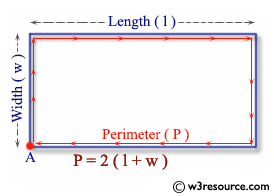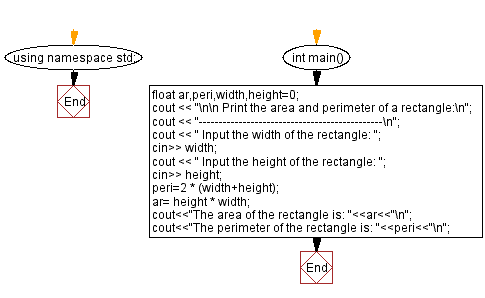﻿ C++ Exercises: Print the area and perimeter of a rectangle - w3resource

# C++ Exercises: Print the area and perimeter of a rectangle

## C++ Basic: Exercise-40 with Solution

Write a C++ program to print the area and perimeter of a rectangle.

Pictorial Presentation:Sample Solution:

C++ Code :

``````#include <iostream>
using namespace std;

int main()
{
float ar,peri,width,height=0;
cout << "\n\n Print the area and perimeter of a rectangle:\n";
cout << "----------------------------------------------\n";
cout << " Input the width of the rectangle: ";
cin>> width;
cout << " Input the height of the rectangle: ";
cin>> height;
peri=2 * (width+height);
ar= height * width;
cout<<"The area of the rectangle is: "<<ar<<"\n";
cout<<"The perimeter of the rectangle is: "<<peri<<"\n";

}
``````

Sample Output:

```  Print the area and perimeter of a rectangle:
----------------------------------------------
Input the width of the rectangle: 8.5
Input the height of the rectangle: 5.6
The area of the rectangle is: 47.6
The perimeter of the rectangle is: 28.2
```

Flowchart:C++ Code Editor:

What is the difficulty level of this exercise?

﻿

## C++ Programming: Tips of the Day

Why is there no std::stou?

The most pat answer would be that the C library has no corresponding "strtou", and the C++11 string functions are all just thinly veiled wrappers around the C library functions: The std::sto* functions mirror strto*, and the std::to_string functions use sprintf.

Ref: https://bit.ly/3wtz2qA

We are closing our Disqus commenting system for some maintenanace issues. You may write to us at reach[at]yahoo[dot]com or visit us at Facebook